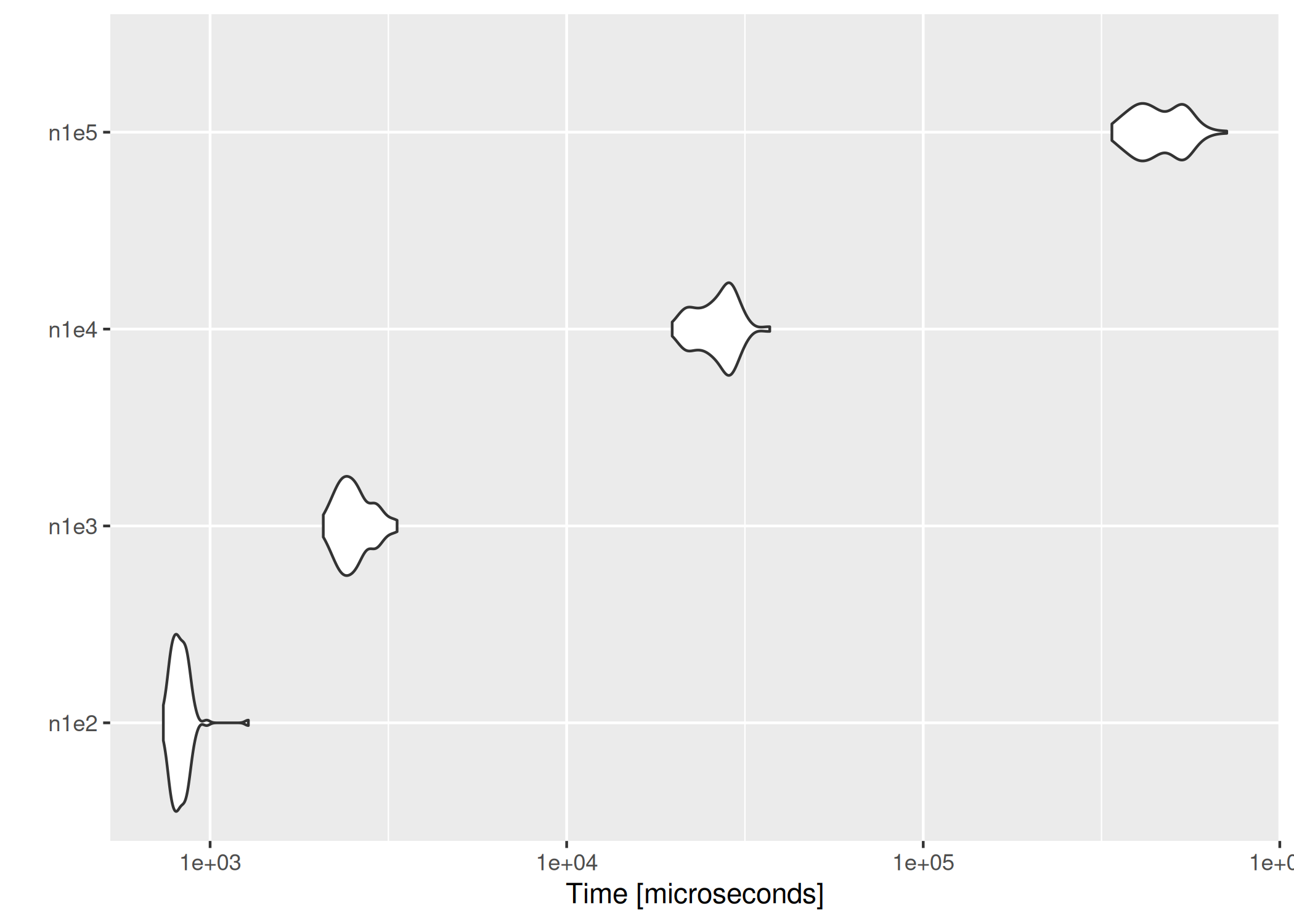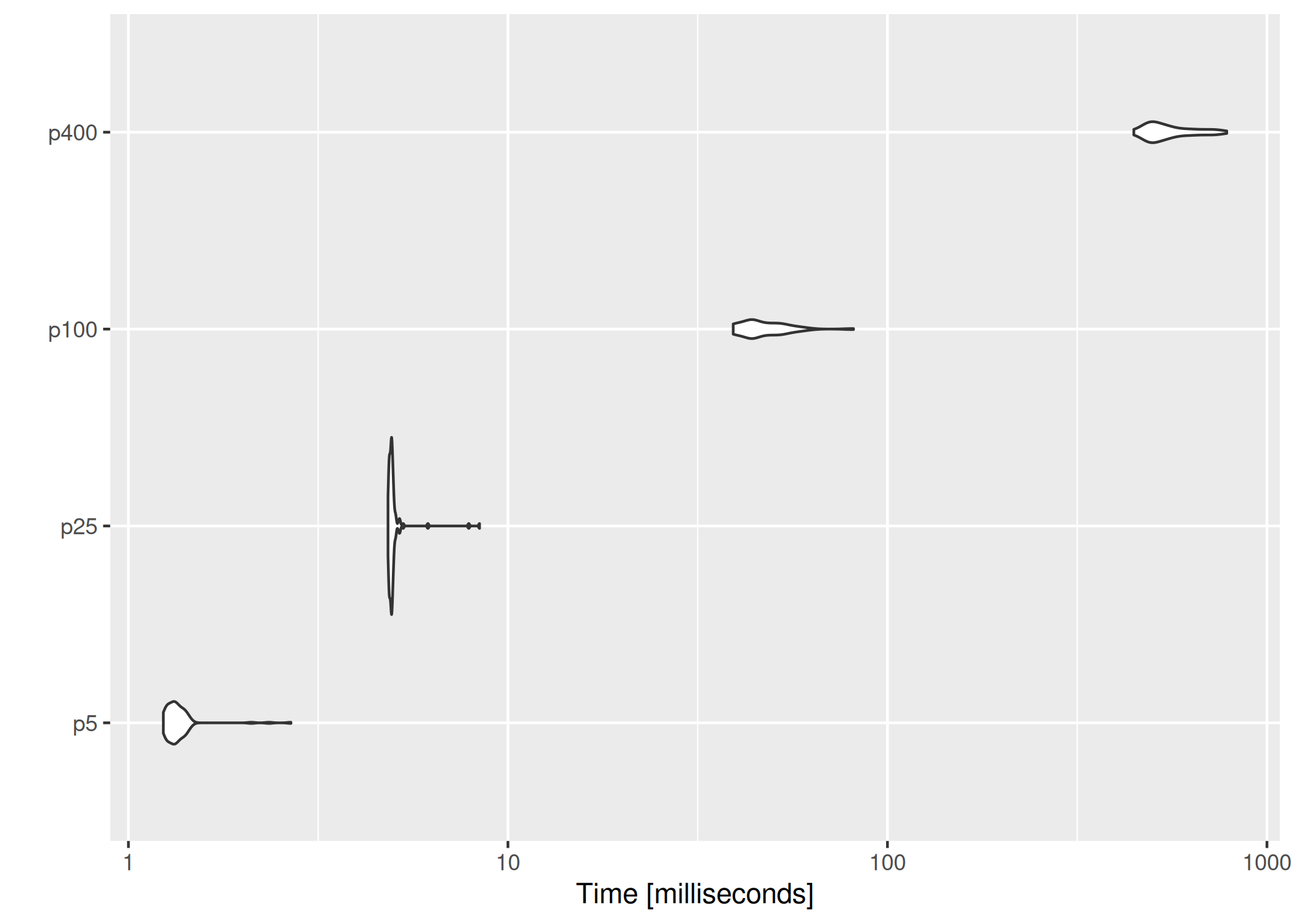# Performance

All the tests were done on an Arch Linux x86_64 machine with an Intel(R) Core(TM) i7 CPU (1.90GHz). We first load the necessary packages.

library(melt)
library(microbenchmark)
library(ggplot2)

## Empirical likelihood computation

We show the performance of computing empirical likelihood with el_mean(). We test the computation speed with simulated data sets in two different settings: 1) the number of observations increases with the number of parameters fixed, and 2) the number of parameters increases with the number of observations fixed.

### Increasing the number of observations

We fix the number of parameters at $$p = 10$$, and simulate the parameter value and $$n \times p$$ matrices using rnorm(). In order to ensure convergence with a large $$n$$, we set a large threshold value using el_control().

set.seed(3175775)
p <- 10
par <- rnorm(p, sd = 0.1)
ctrl <- el_control(th = 1e+10)
result <- microbenchmark(
n1e2 = el_mean(matrix(rnorm(100 * p), ncol = p), par = par, control = ctrl),
n1e3 = el_mean(matrix(rnorm(1000 * p), ncol = p), par = par, control = ctrl),
n1e4 = el_mean(matrix(rnorm(10000 * p), ncol = p), par = par, control = ctrl),
n1e5 = el_mean(matrix(rnorm(100000 * p), ncol = p), par = par, control = ctrl)
)

Below are the results:

result
#> Unit: microseconds
#>  expr        min          lq       mean     median          uq        max neval
#>  n1e2    739.344    788.7035    825.313    816.979    851.8345   1280.260   100
#>  n1e3   2076.968   2334.9270   2544.438   2493.985   2693.3650   3344.997   100
#>  n1e4  19767.768  23754.4475  26536.068  27109.935  28998.7000  37116.624   100
#>  n1e5 338185.628 397765.4175 463856.934 446782.174 527553.9385 710010.555   100
#>  cld
#>  a
#>  a
#>   b
#>    c
autoplot(result)### Increasing the number of parameters

This time we fix the number of observations at $$n = 1000$$, and evaluate empirical likelihood at zero vectors of different sizes.

n <- 1000
result2 <- microbenchmark(
p5 = el_mean(matrix(rnorm(n * 5), ncol = 5),
par = rep(0, 5),
control = ctrl
),
p25 = el_mean(matrix(rnorm(n * 25), ncol = 25),
par = rep(0, 25),
control = ctrl
),
p100 = el_mean(matrix(rnorm(n * 100), ncol = 100),
par = rep(0, 100),
control = ctrl
),
p400 = el_mean(matrix(rnorm(n * 400), ncol = 400),
par = rep(0, 400),
control = ctrl
)
)
result2
#> Unit: milliseconds
#>  expr        min         lq       mean     median         uq        max neval
#>    p5   1.235930   1.276739   1.361866   1.324769   1.381810   2.680283   100
#>   p25   4.830289   4.878286   5.015790   4.933391   4.970878   8.417014   100
#>  p100  39.227604  43.593835  47.621764  44.439667  52.253316  81.381684   100
#>  p400 445.862598 491.519903 555.848706 530.848306 611.759095 783.564377   100
#>  cld
#>  a
#>  a
#>   b
#>    c
autoplot(result2)On average, evaluating empirical likelihood with a 100000×10 or 1000×400 matrix at a parameter value satisfying the convex hull constraint takes less than a second.